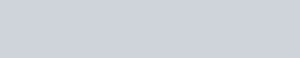Ohms Law and HVAC - Ohms Law is used by HVAC Technicians, electricians, electronics technicians, engineers, and others who deal with electrical circuits every day. It is a necessary fundamental that anyone dealing with electricity or electronics should learn. Ohms law is used in many different applications to solve problems (troubleshooting electrical problems), for sizing wire and designing circuits, and for designing printed circuit boards (PCBs). Ohms law is a basic fundamental formula that helps electrical specialist solves problems. Ohms Law is necessary to learn if you are going to be an above-average technician in HVAC.

Ohms Law, named after physicist Georg Ohm, came about by experimentation by Mr. Ohm. Ohm was fascinated by an invention by the Italian scientist Alessandro Volta, the electrochemical cell. Georg Ohm invented his own instruments to experiment with the new invention which he used to measure volts and current through different lengths of wire. His experimentation led to the discovery of resistance in electrical circuits. Thus, the measurement of resistance in electrical circuits is measured in Ohms using an Ohm meter or by calculating it using Ohms law.

### Ohms Law and HVAC - Ohms Law Formula Applied to HVAC

Sometimes in troubleshooting circuits, an HVAC technician will need to apply Ohms law to their work to solve problems with basic electricity. Take the time to learn this basic electrical law and Ohm’s Law will help you solve problems when troubleshooting HVAC problems especially in HVAC Controls.Current is equal to Voltage divided by the resistance. With this Ohms law formula, one only needs two of the three to calculate the third. You can have the resistance and the current and figure out the voltage. You can have the resistance and the voltage and figure out the current or as stated about you can have the current and the voltage to calculate the resistance. Many technicians use the following diagram to help them remember Ohms law even though it is a simple formula to remember:

Ohms Law and HVAC

The Ohms Law circle above represents the following formulas:
I = E/R or R = E/I or E = I X R
Ohms Law Acronym Definitions:

E = Volts or Electromotive force. Electromotive force is another way of saying volts. Volts are a measurement of electromotive force.

I = Current or Amperes. Amperes or amps are a measurement of current.

R= Resistance or Ohms. Ohms are a measurement of resistance.Ohms Law and HVAC